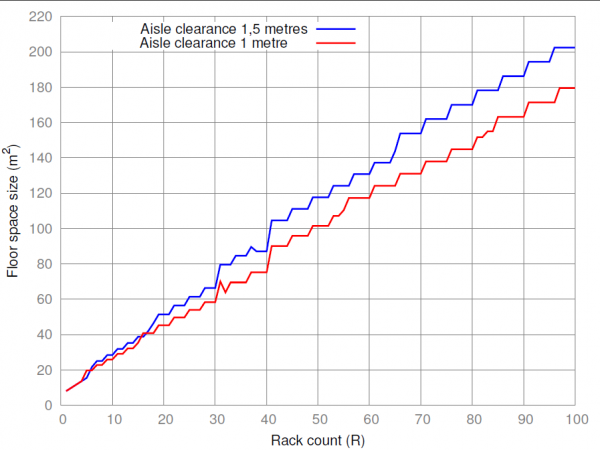# Floorplanning

If you are designing a supercomputer or a data centre, you might be wondering how much floor space you will need, given the specified number of racks with equipment.

Every rack has two dimensions on the floor — its width w and depth d. For a de-facto standard rack, d is about 1,1 metres and w is 0,6 metres. But for IBM’s iDataPlex racks, these dimensions are swapped: d=0,6 m and w=1,2 m.

There must also be clearances around racks so that: (a) you can move racks if necessary, (b) you can easily extract faulty hardware from the racks, and (c) there is good airflow through the rack. You might imagine that you can plan your machine room in such a way that moving racks will never be required, and this could save you some space, but in fact generous clearances are still stipulated by racks manufacturers, so if you want to comply with their recommendations, you will need to keep clearances sufficiently large. See, for example, this drawing for the APC AR3100 rack.

We will denote front clearance (in front of the rack and behind it) with cf, side clearance with cs, and aisle width with ca. A typical arrangement of racks on the floor will then look like this (click to enlarge):

Now, if all dimensions have been specified, and we also know the number of racks to be placed, how do we calculate machine room size? We need an algorithm to place racks on the floor in such a way that the resulting floor space is minimised.

There is also one intricate detail: rack rows cannot be too long. You can’t have a 100 metre long row, because when servicing a rack in the middle of this row you would need to travel a long distance just to get to the back side of the rack. Segment length lxc of six metres — or 10 standard racks — can be a good compromise between convenience and waisted space.

Cluster design tools include a module that allows to place racks on the floor in a square shape, taking into accounts rack dimensions, clearances and the maximal length of rows that you specify. The algorithm for this module solves a quadratic equation and outputs lx and ly, machine room dimensions, as well as a formula for placing racks. The formula describes placement of racks into segments so that you don’t get too long rows.

Default rack dimensions and clearances are as follows: rack width w=0,6 m, depth d=1,2 m, front clearance, side clearance and aisle width are all equal to 1 metre: cf = cs = ca = 1 m, and the maximal length of a contiguous block of racks is 6 metres.

Example 1. For R=200 racks, the algorithm calculates the following machine room dimensions: lx = 19 m, ly = 18,6 m (that is, almost a square). The rack placement formula is (2*9+7)*8, which means that each row has two long segments with 9 racks and one short segment with 7 racks, and there are 8 rows in the room. This alignment gives exactly 200 racks.

Example 2. For R=2,048 racks, the dimensions are lx = 56,4 m and ly = 58,2 m, and the placement formula is (7*10+9)*26. This results in 2,054 racks, which is slightly higher than the original requirement of 2,048 racks.

To make engineering estimates of the required floor space for up to R=100 racks, use the graph below, choosing your preferred aisle width of 1 or 1,5 metres:Floor space size for up to R=100 racks, with aisle clearances of 1 and 1,5 metres.

To use the tool, download and run the distribution, and when the web browser starts, use the link “Floor planning: Calculate floorspace size” (number 1) on the main page. Fill in the number of racks that you need to place, and optionally tune the clearances (number 2):

The output is in a machine-readable format:

```c_a=1.0
c_f=1.0
c_s=1.0
d=1.2
floor_space_plan_formula=(9+8)*6
floor_space_size=187.44
floor_space_x_dimension=13.2
floor_space_y_dimension=14.2
gaps=1
l_xc=6.0
racks=100
racks_per_row=17
rows=6
segments=2
w=0.6```

Machine room dimensions and the placement formula are highlighted in red.

You can download the drawing above in SVG format, best opened with Inkscape.